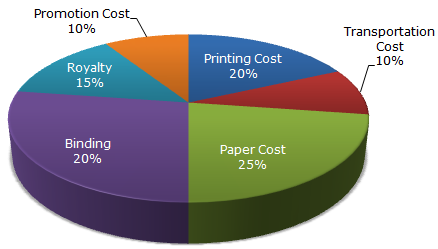# Data Interpretation - Pie Charts

### Exercise :: Pie Charts - Pie Chart 1

The following pie-chart shows the percentage distribution of the expenditure incurred in publishing a book. Study the pie-chart and the answer the questions based on it.

Various Expenditures (in percentage) Incurred in Publishing a Book6.

If the difference between the two expenditures are represented by 18° in the pie-chart, then these expenditures possibly are

 A. Binding Cost and Promotion Cost B. Paper Cost and Royalty C. Binding Cost and Printing Cost D. Paper Cost and Printing Cost

Explanation:

 Central angle of 18° =18 x 100% of the total expenditure 360

= 5% of the total expenditure.

From the given chart it is clear that:

Out of the given combinations, only in combination (d) the difference is 5% i.e.

 Paper Cost - Printing Cost = (25% - 20%) of the total expenditure = 5% of the total expenditure.

7.

For an edition of 12,500 copies, the amount of Royalty paid by the publisher is Rs. 2,81,250. What should be the selling price of the book if the publisher desires a profit of 5%?

 A. Rs. 152.50 B. Rs. 157.50 C. Rs. 162.50 D. Rs. 167.50

Explanation:

Clearly, S.P. of the book = 105% of C.P.

Let the selling price of this edition (of 12500 books) be Rs. x.

 Then, 15 : 105 = 281250 : xx = Rs.105 x 281250= Rs. 1968750. 15S.P. of one book = Rs.1968750= Rs. 157.50 . 12500

8.

If for an edition of the book, the cost of paper is Rs. 56250, then find the promotion cost for this edition.

 A. Rs. 20,000 B. Rs. 22,500 C. Rs. 25,500 D. Rs. 28,125

Explanation:

Let the Promotion Cost for this edition be Rs. p.

 Then, 25 : 10 = 56250 : pp = Rs.56250 x 10= Rs. 22,500. 25

9.

Which two expenditures together have central angle of 108°?

 A. Biding Cost and Transportation Cost B. Printing Cost and Paper Cost C. Royalty and Promotion Cost D. Binding Cost and Paper Cost

Explanation:

 Central angle of 108° =108 x 100% of the total expenditure 360

= 30% of the total expenditure.

From the pie chart it is clear that:

 Binding Cost + Transportation Cost = (20% + 10%) of the total expenditure = 30% of the total expenditure.Binding Cost and Transportation Cost together have a central angle of 108°.